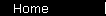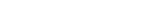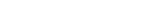# Graham-Style Formula

Benjamin Graham was known for his thorough financial analysis of companies, but he also experimented with many simple rules of thumb. Here is a valuation formula adapted from The Intelligent Investor:

P/E   =   8.5 + 2G
where P/E is the fair P/E ratio, and G is the earnings growth rate.

The idea is that you get a formula that's simple enough to use in the privacy of your own skull, without needing a computer or calculator.

There is a drawback with this formula: as written, it gives answers that are on the high side. But the good news is that with some modifications, this style formula can give you a very fair estimate of the real answer. It's almost as simple as the PEG ratio, and much more accurate.

This calculator lets you check the accuracy of the formula. (It's initialized with a more conservative version of the formula than what's given above.)

 Graham-Style Calculator
Earnings growth rate ("G"):   %
Formula to use:     P/E   =     +   x G

 Results
Fair P/E Ratio:
Graham Formula:

(The "exact answer" depends on growth assumptions specified below.)

Now for something neat-o, the feature we're calling Artificial Ben-telligence (tm). This next calculator takes your growth assumptions and then gives you a Graham-style formula to fit. It's like having Graham reduce your entire belief system to something that could be memorized by a little kid.

 Assumptions
The growth rate "G" will be sustained for the next years,
before leveling off to an annual growth rate of % thereafter.

The discount rate is %

 Results
Formula to use:     P/E   =     +   x G
Carry formula to top calculator to check its accuracy . . .

To use this tool, first choose realistic growth assumptions (being conservative - remember that you're trying to protect yourself from overpaying) and memorize the simple formula that comes out of the second calculator. Use the first calculator to see what values of "G" the formula works well for (huge "G" and/or aggressive growth assumptions are what make it fail). Then you can amaze financial experts with your freakish ability to estimate a two-stage discounted cash flow analysis in your head.

home  |  article  |  glossary  |  calculator  |  about us  |  booksValuation IntroA Little TheoryDCF CalculatorP/E RatioP/S RatioPEG RatioGraham FormulaDividend DiscountBuffett Formula (?)CAPM CalculatorBooks & LinksValuation FormulaStock Market PredictionsMeasuring Investment ReturnsStock Market CAGR